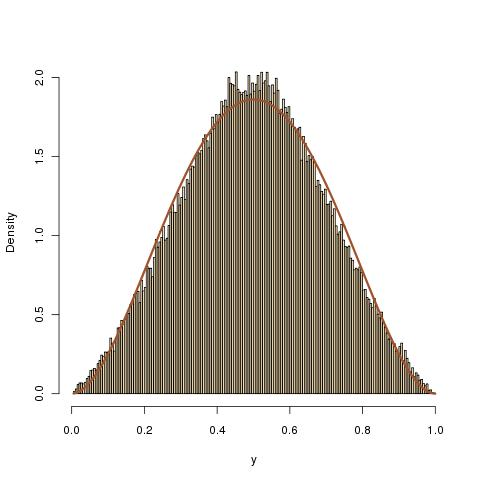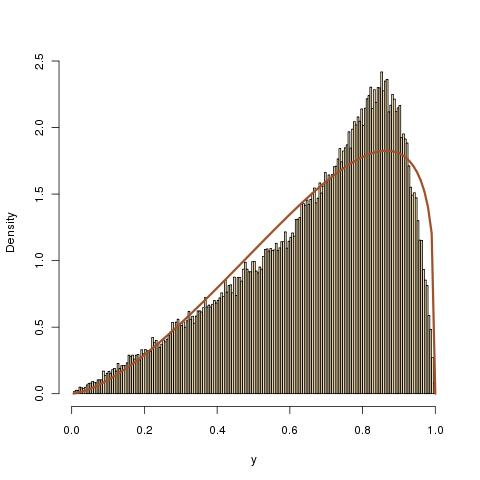Want to share your content on R-bloggers? click here if you have a blog, or here if you don't.

On his Probability and statistics blog, Matt Asher put a funny question (with my rephrasing):

Take a unit square. Now pick two spots at random along the perimeter, uniformly. For each of these two locations, pick another random point from one of the three other sides of the square and draw the segment. What is the probability the two segments intersect? And what is the distribution for the intersection points?

The (my) intuition for the first question was 1/2, but a quick computation led to another answer. The key to the computation is to distinguish whether or not both segments share one side of the square. They do with probability$dfrac{2}{4}times 1 + dfrac{2}{4}timesdfrac{2}{3} = dfrac{5}{6},$

in which case they intersect with probability 1/2. They occupy the four sides with probability 1/6, in which case they intersect with probability 1/3. So the final answer is 17/36 (as posted by several readers and empirically found by Matt). The second question is much more tricky: the histogram of the distribution of the coordinates is peaked towards the boundaries, thus reminding me of an arc-sine distribution, but there is a bump in the middle as well. Computing the coordinates of the intersection depending on the respective positions of the endpoints of both segments and simulating those distributions led me to histograms that looked either like beta B(a,a) distributions, or like beta B(1,a) distributions, or like beta B(a,1) distributions… Not exactly, though. So not even a mixture of beta distributions is enough to explain the distribution of the intersection points… For instance, the intersection points corresponding to segments were both segments start from the same side and end up in the opposite side are distributed as$dfrac{u_1(u_4-u_3)-u_3(u_2-u_1)}{u_4-u_3-u_2+u_1}$

where all u‘s are uniform on (0,1) and under the constraint$(u_2-u_1)(u_4-u_3)<0$. The following graph shows how well a beta distribution fits in that case. (Not perfectly, though!)The R code is

u=matrix(runif(4*10^5),ncol=4)
u[,c(1,3)]=t(apply(u[,c(1,3)],1,sort))
u[,c(2,4)]=-t(apply(-u[,c(2,4)],1,sort))
y=(u[,1]*(u[,4]-u[,3])-u[,3]*(u[,2]-u[,1]))/(u[,1]+u[,4]-u[,2]-u[,3])

Similarly, if the two segments start from the same side but end up on different sides, the distribution of one coordinate is given by$dfrac{u_1(1-u_3)-u_3u_4(u_2-u_1)}{1-u_3-u_4(u_2-u_1)}$

under the constraint$u_3. The outcome is once again almost distributed as a beta:The corresponding R code is

u=matrix(runif(4*10^5),ncol=4)
u[,c(1,3)]=-t(apply(-u[,c(1,3)],1,sort))
y=(u[,1]*(1-u[,3])-u[,3]*u[,4]*(u[,2]-u[,1]))/(1-u[,3]-u[,4]*(u[,2]-u[,1]))